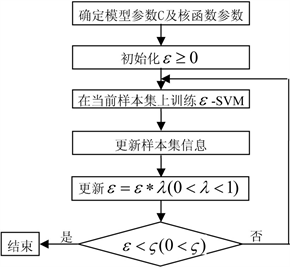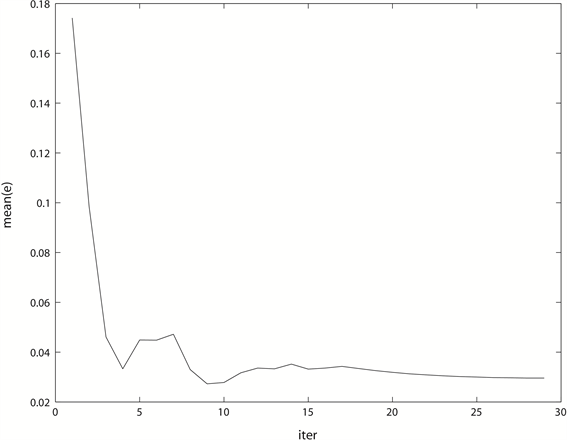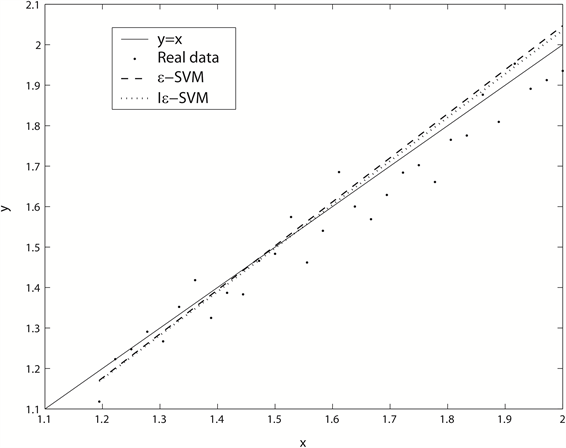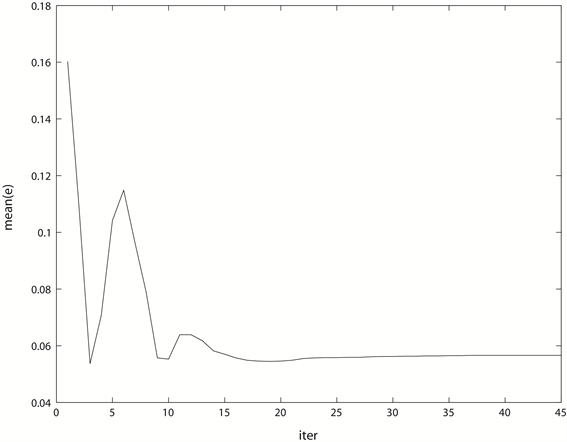# 基于迭代的支持向量机的产品销售预测模型Product Sale Forecasting Model Based on Iterative Support Vector Machine

• 全文下载: PDF(814KB)    PP.60-69   DOI: 10.12677/CSA.2020.101008
• 下载量: 52  浏览量: 86   国家自然科学基金支持

Aiming at data characteristics of small samples and noise existing in the product sale series, an iterative support vector machine (Iε-SVM) is proposed in this paper. During the gradually reducing process of Iε-SVM’s parameter ε, the samples greatly affected by noise are iteratively amended to reduce their influence on the final forecasting model generated. Iε-SVM is applied to a numerical value example and the automobile sales forecasting in contrast with the ε support vector machine (ε-SVM). The experiment results indicate that Iε-SVM is effective and feasible, by which more accurate forecasting results are obtained over the ε-SVM.

1. 引言

2. 支持向量机

$\begin{array}{l}\underset{w,b,{\xi }^{\left(\ast \right)}}{min}\text{ }\tau \left(w,b,{\xi }^{\left(\ast \right)}\right)=\frac{1}{2}{‖w‖}^{2}+C\underset{i=1}{\overset{l}{\sum }}\left({\xi }_{i}+{\xi }_{i}^{\ast }\right)\\ \text{s}\text{.t}\text{.}\left\{\begin{array}{l}{y}_{i}-\left(w\cdot {x}_{i}+b\right)\le \epsilon +{\xi }_{i}\\ \left(w\cdot {x}_{i}+b\right)-{y}_{i}\le \epsilon +{\xi }_{i}^{*}\\ {\xi }^{\left(\ast \right)}\ge 0\end{array}\end{array}$ (1)

$\begin{array}{l}\underset{\alpha ,{\alpha }^{*}}{\mathrm{min}}\text{ }W\left(\alpha ,{\alpha }^{*}\right)=\frac{1}{2}\underset{i,j=1}{\overset{l}{\sum }}\left({\alpha }_{i}^{*}-{\alpha }_{i}\right)\left({\alpha }_{j}^{*}-{\alpha }_{j}\right)k\left({x}_{i},{x}_{j}\right)\\ \text{\hspace{0.17em}}\text{\hspace{0.17em}}\text{\hspace{0.17em}}\text{\hspace{0.17em}}\text{\hspace{0.17em}}\text{\hspace{0.17em}}\text{\hspace{0.17em}}\text{\hspace{0.17em}}\text{\hspace{0.17em}}\text{\hspace{0.17em}}\text{\hspace{0.17em}}\text{\hspace{0.17em}}\text{\hspace{0.17em}}\text{\hspace{0.17em}}\text{\hspace{0.17em}}\text{\hspace{0.17em}}\text{\hspace{0.17em}}\text{\hspace{0.17em}}\text{\hspace{0.17em}}\text{\hspace{0.17em}}\text{\hspace{0.17em}}\text{\hspace{0.17em}}\text{\hspace{0.17em}}\text{\hspace{0.17em}}\text{\hspace{0.17em}}-\underset{i=1}{\overset{l}{\sum }}\left({\alpha }_{i}^{*}-{\alpha }_{i}\right){y}_{i}+\underset{i=1}{\overset{l}{\sum }}\left({\alpha }_{i}^{*}+{\alpha }_{i}\right)\epsilon \\ \text{s}\text{.t}.\left\{\begin{array}{l}\underset{i=1}{\overset{l}{\sum }}\left({\alpha }_{i}^{*}-{\alpha }_{i}\right)=0\\ {\alpha }_{i},{\alpha }_{i}^{*}\in \left[0,C\right],i=1,\cdots ,l\end{array}\end{array}$ (2)

$f\left(x\right)=\underset{i=1}{\overset{l}{\sum }}\left({\alpha }_{i}^{\ast }-{\alpha }_{i}\right)k\left({x}_{i},x\right)+b$ (3)

3. 迭代支持向量机(Iε-SVM)

ε-SVM采用ε不敏感函数，夹在曲线 $f\left(x\right)=\underset{i=1}{\overset{l}{\sum }}\left({\alpha }_{i}^{\ast }-{\alpha }_{i}\right)k\left({x}_{i},x\right)+b+\epsilon$$f\left(x\right)=\underset{i=1}{\overset{l}{\sum }}\left({\alpha }_{i}^{\ast }-{\alpha }_{i}\right)k\left({x}_{i},x\right)+b-\epsilon$ 中间的区域被称为ε-带，当样本点位于ε-带中时则认为模型在该点没有损失，只有当样本点位于ε-带之外时，才有损失出现。针对样本点 $\left({x}_{i},{y}_{i}\right)$ 和ε-带之间的位置关系，我们有下面的定理。

i) 若 ${\alpha }_{i}={\alpha }_{i}^{*}=0$，则相应的样本点 $\left({x}_{i},{y}_{i}\right)$ 一定在ε-带的内部或边界上。

ii) 若 ${\alpha }_{i}\in \left(0,C\right),{\alpha }_{i}^{*}=0$${\alpha }_{i}=0,{\alpha }_{i}^{*}\in \left(0,C\right)$，则相应的样本点 $\left({x}_{i},{y}_{i}\right)$ 一定在ε-带的边界上。

iii) 若 ${\alpha }_{i}=C,{\alpha }_{i}^{*}=0$${\alpha }_{i}=0,{\alpha }_{i}^{*}=C$，则相应的样本点 $\left({x}_{i},{y}_{i}\right)$ 一定在ε-带的外部或边界上。

${\alpha }_{i}\left(\epsilon +{\xi }_{i}-{y}_{i}+w\cdot {x}_{i}+b\right)=0$ (4)

${\alpha }_{i}^{*}\left(\epsilon +{\xi }_{i}+{y}_{i}-w\cdot {x}_{i}-b\right)=0$ (5)

$\left(C-{\alpha }_{i}\right){\xi }_{i}=0$ (6)

$\left(C-{\alpha }_{i}^{*}\right){\xi }_{i}^{*}=0$ (7)

i) 由式(6)和(7)可以看出 $\xi ={\xi }_{i}^{*}=0$

ii) 由式(6)和(7)可以看出 $\xi ={\xi }_{i}^{*}=0$，同时结合式(4)和(5)可断定，当 ${\alpha }_{i}\ne 0$ 时， $\epsilon +{\xi }_{i}-{y}_{i}+w\cdot {x}_{i}+b=0$ ；当 ${\alpha }_{i}^{*}\ne 0$ 时， $\epsilon +{\xi }_{i}+{y}_{i}-w\cdot {x}_{i}-b=0$

iii) 由式(4)~(7)可以看出。Figure 1. The flow chart of Iε-SVM

Iε-SVM的运行机制如图1所示，我们首先确定模型参数C及可能存在的核函数参数，为ε选取一个较大的初始值，训练ε-SVM，更新落入ε-带外部的样本点 $\left({x}_{i},{y}_{i}\right)$ 的值， $\lambda$ 是个预先给定的比例系数，每次按比例 $\lambda$ 缩小ε； $\varsigma$ 是一个预先给定的阀值，如果 $\epsilon <\varsigma$ 则终止算法，否则更新ε的值，并在更新后的样本集上训练ε-SVM。

1) 若 ${\alpha }_{i}\ne C$${\alpha }_{i}^{*}\ne C$，此时样本点 $\left({x}_{i},{y}_{i}\right)$ ε-带的内部或边界上，则令 ${\stackrel{˜}{y}}_{i}={y}_{i}$

2) 若 ${\alpha }_{i}=C,{\alpha }_{i}^{*}=0$，此时 ${y}_{i}\ge w\cdot {x}_{i}+b+\epsilon$，我们令 ${\stackrel{˜}{y}}_{i}=w\cdot {x}_{i}+b+\epsilon$

3) 若 ${\alpha }_{i}=0,{\alpha }_{i}^{*}=C$，此时 ${y}_{i}\le w\cdot {x}_{i}+b-\epsilon$，我们令 ${\stackrel{˜}{y}}_{i}=w\cdot {x}_{i}+b-\epsilon$

$\begin{array}{l}\underset{w,b,{\xi }^{\left(\ast \right)}}{min}\text{ }\tau \left(w,b,{\xi }^{\left(\ast \right)}\right)=\frac{1}{2}{‖w‖}^{2}+C\underset{i=1}{\overset{l}{\sum }}\left({\xi }_{i}+{\xi }_{i}^{\ast }\right)\\ \text{s}\text{.t}\text{.}\left\{\begin{array}{l}{\stackrel{˜}{y}}_{i}-\left(w\cdot {x}_{i}+b\right)\le \epsilon +{\xi }_{i}\\ \left(w\cdot {x}_{i}+b\right)-{\stackrel{˜}{y}}_{i}\le \epsilon +{\xi }_{i}^{*}\\ {\xi }^{\left(\ast \right)}\ge 0\end{array}\end{array}$ (8)

i) ${\stackrel{˜}{y}}_{i}={y}_{i}$，此时 $\left({x}_{i},{y}_{i}\right)$ 落入曲线 $y=\stackrel{¯}{w}\cdot x+\stackrel{¯}{b}$ 的ε-带内部或边界上，有：

${\stackrel{˜}{y}}_{i}-\left(\stackrel{¯}{w}\cdot {x}_{i}+\stackrel{¯}{b}\right)\le \epsilon ,$

$\left(\stackrel{¯}{w}\cdot {x}_{i}+\stackrel{¯}{b}\right)-{\stackrel{˜}{y}}_{i}\le \epsilon .$

ii) ${\stackrel{˜}{y}}_{i}=\stackrel{¯}{w}\cdot {x}_{i}+\stackrel{¯}{b}+\epsilon$，此时 $\left({x}_{i},{y}_{i}\right)$ 落入曲线 $y=\stackrel{¯}{w}\cdot x+\stackrel{¯}{b}$ 的ε-带外部或边界上，有：

${\stackrel{˜}{y}}_{i}-\left(\stackrel{¯}{w}\cdot {x}_{i}+\stackrel{¯}{b}\right)\le \epsilon ,$

$\left(\stackrel{¯}{w}\cdot {x}_{i}+\stackrel{¯}{b}\right)-{\stackrel{˜}{y}}_{i}\le \epsilon .$

iii) ${\stackrel{˜}{y}}_{i}=\stackrel{¯}{w}\cdot {x}_{i}+\stackrel{¯}{b}-\epsilon$，此时 $\left({x}_{i},{y}_{i}\right)$ 同样落入曲线 $y=\stackrel{¯}{w}\cdot x+\stackrel{¯}{b}$ 的ε-带外部或边界上，有：

${\stackrel{˜}{y}}_{i}-\left(\stackrel{¯}{w}\cdot {x}_{i}+\stackrel{¯}{b}\right)\le \epsilon ,$

$\left(\stackrel{¯}{w}\cdot {x}_{i}+\stackrel{¯}{b}\right)-{\stackrel{˜}{y}}_{i}\le \epsilon .$

$\tau \left(\stackrel{¯}{w},\stackrel{¯}{b},0\right)\ge \tau \left(\stackrel{˜}{w},\stackrel{˜}{b},{\stackrel{˜}{\xi }}^{\left(\ast \right)}\right),$

$\frac{1}{2}{‖\stackrel{˜}{w}‖}^{2}+C\underset{i=1}{\overset{l}{\sum }}\left({\stackrel{˜}{\xi }}_{i}+{\stackrel{˜}{\xi }}_{i}^{\ast }\right)\le \frac{1}{2}{‖\stackrel{¯}{w}‖}^{2}$，而 ${\stackrel{˜}{\xi }}^{\left(\ast \right)}\ge 0$，所以有：

${‖\stackrel{˜}{w}‖}^{2}\le {‖\stackrel{¯}{w}‖}^{2}.$

4. 仿真实验

4.1. 比较准则

${e}_{i}=|{y}_{i}-{{y}^{\prime }}_{i}|,$ (9)

1) 最大预测误差( $\mathrm{max}\left(e\right)\right)$

$\mathrm{max}\left(e\right)=\underset{1\le i\le {l}_{2}}{\mathrm{max}}\left({e}_{i}\right),$ (10)

2) 最小预测误差( $\mathrm{min}\left(e\right)\right)$

$\mathrm{min}\left(e\right)=\underset{1\le i\le {l}_{2}}{\mathrm{min}}\left({e}_{i}\right)$ (11)

3) 平均预测误差( $\text{mean}\left(e\right)\right)$

$\text{mean}\left(e\right)=\frac{1}{{l}_{2}}\left(\underset{i=1}{\overset{{l}_{2}}{\sum }}{e}_{i}\right)$ (12)

4) 预测误差方差( $\mathrm{var}\left(e\right)\right)$

$\mathrm{var}\left(e\right)=\frac{1}{{l}_{2}-1}\underset{i=1}{\overset{{l}_{2}}{\sum }}{\left({e}_{i}-\text{mean}\left(e\right)\right)}^{2}$ (13)

4.2. 数值算例

$y=x+\xi ,$ (14)

$\xi$ 是服从 $N\left(0,0.05\right)$ 的随机数，x分别取区间 $\left[1,2\right]$ 中平均分布的36个节点，并通过式14计算相应的y值，产生36组数据 $\left(x,y\right)$ 构成样本集T。将样本集T分为训练样本集 ${T}_{1}$ 和测试样本集 ${T}_{2}$ 两个部分，训练样本集 ${T}_{1}$ 取样本集T得前 ${l}_{1}$ 个样本，余下样本作为测试样本集 ${T}_{2}$${l}_{1}$ 分别取6、12、24三种不同情况，以便比较两种模型在不同规模训练样本集下的表现。分别将ε-SVM与Iε-SVM作用于训练样本集 ${T}_{1}$ 建立模型，ε-SVM与Iε-SVM采用相同的核函数：

$k\left({x}_{i},{x}_{j}\right)={x}_{i}\cdot {x}_{j}$Table 1. The forecasting errors of two models in the numerical experimentFigure 2. The decline curve of the average forecasting error of Iε-SVM in iteration when ${l}_{1}=6$Figure 3. The forecasting results of two models when ${l}_{1}=6$

4.3. 应用实例

$K\left({x}_{i},{x}_{j}\right)=\mathrm{exp}\left(-\frac{{‖{x}_{i}-{x}_{j}‖}^{2}}{{\sigma }^{2}}\right)$Figure 4. The decline curve of the average forecasting error of Iε-SVM in iterationFigure 5. The forecasting results of two modelsTable 2. The forecasting errors of two models in the practical experiment

5. 结论

  Vapnik, V.N. (2000) The Nature of Statistical Learning Theory. Springer, New York, 138-167. https://doi.org/10.1007/978-1-4757-3264-1  邓乃扬, 田英杰. 数据挖掘中的新方法—支持向量机[M]. 北京: 科学出版社, 2006.  周辉仁, 郑丕谔, 任仙玲. 最小二乘支持向量机的参数优选方法及应用[J]. 系统工程学报, 2009, 24(2): 248-252.  姚宝珍, 杨成永, 于滨. 动态公交车辆运行时间预测模型[J]. 系统工程学报, 2010, 25(3): 365-370.  李大铭, 于滨. 公交运营的协控准点滞站调度模型[J]. 系统工程学报, 2012, 27(2): 248-255.  商志根, 严洪森. 基于模糊支持向量机的产品设计时间预测[J]. 控制与决策, 2012, 27(4): 531-534.  时培明, 梁凯, 赵娜, 等. 基于深度学习特征提取和粒子群支持向量机状态识别的齿轮智能故障诊断[J]. 中国机械工程, 2017, 28(9): 1056-1061.  张燕君, 王会敏, 付兴虎, 等. 基于粒子群支持向量机的钢板损伤位置识别[J]. 中国激光, 2017, 44(10): 197-203.  肖鹏飞, 张超勇, 罗敏, 等. 基于自适应动态无偏最小二乘支持向量机的刀具磨损预测建模[J]. 中国机械工程, 2018, 29(7): 842-849.  张英, 苏宏业, 褚健. 基于模糊最小二乘支持向量机的软测量建模[J]. 控制与决策, 2005, 20(6): 621-624.  姚潇, 余乐安. 模糊近似支持向量机模型及其在信用风险评估中的应用[J]. 系统工程理论实践, 2012, 32(3): 549-554.  Lin, C.F. and Wang, S.D. (2004) Training Algorithms for Fuzzy Support Vector Machines with Noisy Data. Pattern Recognition Let-ters, 25, 1647-1656. https://doi.org/10.1016/j.patrec.2004.06.009  Li, D., Hu, W.C., Xiong, W. and Yang, J.B. (2008) Fuzzy Relevance Vector Machine for Learning from Unbalanced Data and Noise. Pattern Recognition Letters, 29, 1175-1181. https://doi.org/10.1016/j.patrec.2008.01.009  张桂香, 费岚, 杜喆, 刘三阳. 非均衡数据的去噪模糊支持向量机新方法[J]. 计算机工程与应用, 2008, 44(16): 142-144.  蒋蔚, 伊国兴, 曾庆双. 一种基于SVM重采样的似然粒子滤波算法[J]. 控制与决策, 2011, 26(2): 243-247.  吴奇, 严洪森. 基于具有高斯损失函数支持向量机的预测模型[J]. 计算机集成制造系统, 2009, 15(2): 306-312.  Li, H.X., Yang, J.L., Zhang, G. and Fan, B. (2013) Probabilistic Support Vector Machines for Classification of Noise Affected Data. Information Sci-ences, 221, 60-71. https://doi.org/10.1016/j.ins.2012.09.041  Keerthi, S.S., Shevade, S.K., Bhattacharyya, C. and Murthy, K.R.K. (2000) A Fast Iterative Nearest Point Algorithm for Support Vector Machine Classifier Design. IEEE Transactions on Neural Networks, 11, 124-136. https://doi.org/10.1109/72.822516  Zhou, S.S., Liu, H.W., Ye, F. and Zhou, L.H. (2009) A New Iterative Al-gorithm Training SVM. Optimization Methods and Software, 24, 913-932. https://doi.org/10.1080/10556780902867906  Ye, Q.L., Zhao, C.X., Ye, N. and Chen, Y.N. (2010) Iterative Support Vector Machine with Guaranteed Accuracy and Run Time. Expert Systems, 27, 338-348. https://doi.org/10.1111/j.1468-0394.2010.00550.x  Liu, D.H., Qian, H., Dai, G. and Zhang, Z. (2013) An Iter-ative SVM Approach to Feature Selection and Classification in High-Dimensional Datasets. Pattern Recognition, 46, 2531-2537. https://doi.org/10.1016/j.patcog.2013.02.007